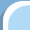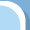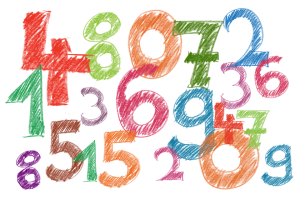sederet.com
Online Indonesian - English DictionaryEnglish to Indonesian Indonesian to English English to English# Angka 1 – 100 dalam Bahasa InggrisBahasa Inggris memiliki dua jenis angka, yakni cardinal numbers dan ordinal numbers. Kali ini kita akan mempelajari angka cardinal dan ordinal 1 sampai 100 dalam bahasa Inggris.

Cardinal numbers

Cardinal numbers adalah angka utama dalam bahasa Inggris yang bisa digunakan untuk menyebutkan jumlah dan berhitung. Berikut adalah cardinal numbers bahasa Inggris 1 sampai 100:

• 1 = One
• 2 = Two
• 3 = Three
• 4 = Four
• 5 = Five
• 6 = Six
• 7 = Seven
• 8 = Eight
• 9 = Nine
• 10 = Ten
• 11 = Eleven
• 12 = Twelve
• 13 = Thirteen
• 14 = Fourteen
• 15 = Fifteen
• 16 = Sixteen
• 17 = Seventeen
• 18 = Eighteen
• 19 = Nineteen
• 20 = Twenty
• 21 = Twenty-one
• 22 = Twenty-two
• 23 = Twenty-three
• 24 = Twenty-four
• 25 = Twenty-five
• 26 = Twenty-six
• 27 = Twenty-seven
• 28 = Twenty-eight
• 29 = Twenty-nine
• 30 = Thirty
• 31 = Thirty-one
• 32 = Thirty-two
• 33 = Thirty-three
• 34 = Thirty-four
• 35 = Thirty-five
• 36 = Thirty-six
• 37 = Thirty-seven
• 38 = Thirty-eight
• 39 = Thirty-nine
• 40 = Forty
• 41 = Forty-one
• 42 = Forty-two
• 43 = Forty-three
• 44 = Forty-four
• 45 = Forty-five
• 46 = Forty-six
• 47 = Forty-seven
• 48 = Forty-eight
• 49 = Forty-nine
• 50 = Fifty
• 51 = Fifty-one
• 52 = Fifty-two
• 53 = Fifty-three
• 54 = Fifty-four
• 55 = Fifty-five
• 56 = Fifty-six
• 57 = Fifty-seven
• 58 = Fifty-eight
• 59 = Fifty-nine
• 60 = Sixty
• 61 = Sixty-one
• 62 = Sixty-two
• 63 = Sixty-three
• 64 = Sixty-four
• 65 = Sixty-five
• 66 = Sixty-six
• 67 = Sixty-seven
• 68 = Sixty-eight
• 69 = Sixty-nine
• 70 = Seventy
• 71 = Seventy-one
• 72 = Seventy-two
• 73 = Seventy-three
• 74 = Seventy-four
• 75 = Seventy-five
• 76 = Seventy-six
• 77 = Seventy-seven
• 78 = Seventy-eight
• 79 = Seventy-nine
• 80 = Eighty
• 81 = Eighty-one
• 82 = Eighty-two
• 83 = Eighty-three
• 84 = Eight-four
• 85 = Eighty-five
• 86 = Eight-six
• 87 = Eighty-seven
• 88 = Eighty-eight
• 89 = Eighty-nine
• 90 = Ninety
• 91 = Ninety-one
• 92 = Ninety-two
• 93 = Ninety-three
• 94 = Ninety-four
• 95 = Ninety-five
• 96 = Ninety-six
• 97 = Ninety-seven
• 98 = Ninety-eight
• 99 = Ninety-nine
• 100 = One hundred/ a hundred

Ordinal numbers

Ordinal numbers adalah angka yang digunakan untuk menyebutkan urutan atau peringkat (misal: pertama, kedua, ketiga, dan sebagainya). Berikut adalah ordinal numbers bahasa Inggris dari ke-1 sampai ke-100:

• 1st = First
• 2nd = Second
• 3rd = Third
• 4th = Fourth
• 5th = Fifth
• 6th = Sixth
• 7th = Seventh
• 8th = Eighth
• 9th = Ninth
• 10th = Tenth
• 11th = Eleventh
• 12th = Twelfth
• 13th = Thirteenth
• 14th = Fourteenth
• 15th = Fifteenth
• 16th = Sixteenth
• 17th = Seventeenth
• 18th = Eighteenth
• 19th = Nineteenth
• 20th = Twentieth
• 21st = Twenty-first
• 22nd = Twenty-second
• 23rd = Twenty-third
• 24th = Twenty-fourth
• 25th = Twenty-fifth
• 26th = Twenty-sixth
• 27th = Twenty-seventh
• 28th = Twenty-eighth
• 29th = Twenty-ninth
• 30th = Thirtieth
• 31st = Thirty-first
• 32nd = Thirty-second
• 33rd = Thirty-third
• 34th = Thirty-fourth
• 35th = Thirty-fifth
• 36th = Thirty-sixth
• 37th = Thirty-seventh
• 38th = Thirty-eighth
• 39th = Thirty-ninth
• 40th = Fortieth
• 41st = Forty-first
• 42nd = Forty-second
• 43rd = Forty-third
• 44th = Forty-fourth
• 45th = Forty-fifth
• 46th = Forty-sixth
• 47th = Forty-seventh
• 48th = Forty-eighth
• 49th = Forty-ninth
• 50th = Fiftieth
• 51st = Fifty-first
• 52nd = Fifty-second
• 53rd = Fifty-third
• 54th = Fifty-fourth
• 55th = Fifty-fifth
• 56th = Fifty-sixth
• 57th = Fifty-seventh
• 58th = Fifty-eighth
• 59th = Fifty-ninth
• 60th = Sixtieth
• 61st = Sixty-first
• 62nd = Sixty-second
• 63rd = Sixty-third
• 64th = Sixty-fourth
• 65th = Sixty-fifth
• 66th = Sixty-sixth
• 67th = Sixty-seventh
• 68th = Sixty-eighth
• 69th = Sixty-ninth
• 70th = Seventieth
• 71st = Seventy-first
• 72nd = Seventy-second
• 73rd = Seventy-third
• 74th = Seventy-fourth
• 75th = Seventy-fifth
• 76th = Seventy-sixth
• 77th = Seventy-seventh
• 78th = Seventy-eighth
• 79th = Seventy-ninth
• 80th = Eightieth
• 81st = Eighty-first
• 82nd = Eighty-second
• 83rd = Eighty-third
• 84th = Eight-fourth
• 85th = Eighty-fifth
• 86th = Eighty-sixth
• 87th = Eighty-seventh
• 88th = Eighty-eighth
• 89th = Eighty-ninth
• 90th = Ninetieth
• 91st = Ninety-first
• 92nd = Ninety-second
• 93rd = Ninety-third
• 94th = Ninety-fourth
• 95th = Ninety-fifth
• 96th = Ninety-sixth
• 97th = Ninety-seventh
• 98th = Ninety-eighth
• 99th = Ninety-ninth
• 100th = One hundredth